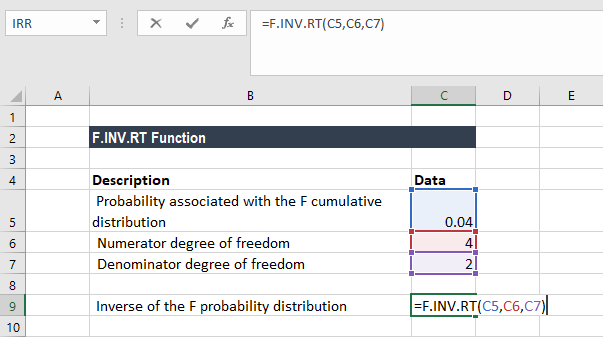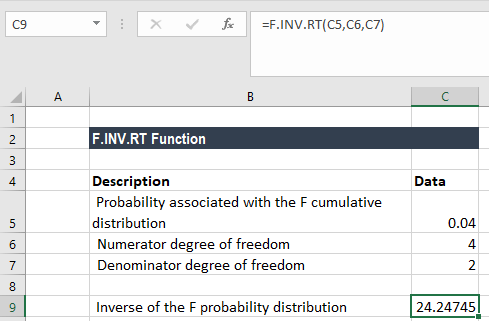# F.INV.RT Function

Calculates the inverse of the (right-tailed) F probability distribution

## What is the F.INV.RT Function?

The F.INV.RT Function is an Excel Statistical function that calculates the inverse of the (right-tailed) F probability distribution.

As a financial analyst, this function is useful in understanding the variability in two data sets. For example, we can use F.INV.RT if we wish to analyze the income diversity between two regions where a company sells its products.

F.INV.RT is an updated version of the previous FINV function that is still available on earlier versions of MS Excel.

### Formula

=F.INV.RT(probability, deg_freedom1, deg_freedom2)

The F.INV.RT function uses the following arguments:

1. Probability (required argument) – This is the probability at which we evaluate the inverse Cumulative F Distribution. The value is between 0 and 1.
2. Deg_freedom1 (required argument) – This is an integer specifying the numerator degrees of freedom.
3. Deg_freedom2 (required argument) – This is an integer specifying the denominator degrees of freedom.

If p = F.DIST.RT(x,…), then F.INV.RT(p,…) = x. Here, we need to remember that the Excel F.INV function is the inverse of the cumulative form of the Excel F.DIST function.

That is, if:

F.DIST(x, deg_freedom1, deg_freedom2, True) = probability

Then,

F.INV(probability, deg_freedom1, deg_freedom2) = x

### How to use the F.INV.RT Function in Excel?

To understand the uses of the F.INV.RT function, let’s consider an example:

#### Worked Step-by-Step Example

Suppose we are given the following data:

• The probability associated with the F cumulative distribution: 04
• Numerator degree of freedom: 4
• Denominator degree of freedom: 2

To find out the inverse of the F probability distribution using the F.INV.RT function, we will use the following formula:We get the result below:### Things to remember about the F.INV.RT Function

1. If either deg_freedom1 or deg_freedom2 is a decimal number, it is truncated to integers by MS Excel.
2. #NUM! error – Occurs if either:
• The probability that is provided is less than or equal to 0 or greater than 1; or
• The argument deg_freedom1 or deg_freedom2 is less than 1.
3. #VALUE! error – Occurs when any of the arguments provided are non-numeric.
4. The function was introduced in MS Excel 2010 and is available in subsequent versions.
5. INV.RT seeks that value x such that F.DIST.RT(x, deg_freedom1, deg_freedom2) = probability. So, the precision of F.INV.RT depends on the precision of F.DIST.RT. F.INV.RT uses an iterative search technique. If the search fails to converge after 64 iterations, the function returns the #N/A error value.

For more information on the function check out its documentation on Excel support website.

Thanks for reading CFI’s guide to important Excel functions! By taking the time to learn and master these functions, you’ll significantly speed up your financial analysis. To learn more, check out these additional CFI resources:

• Excel Functions for Finance